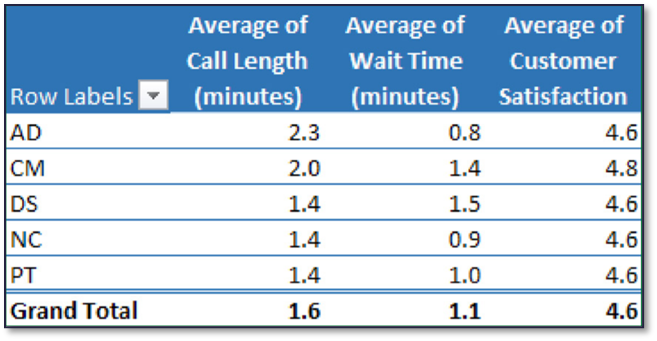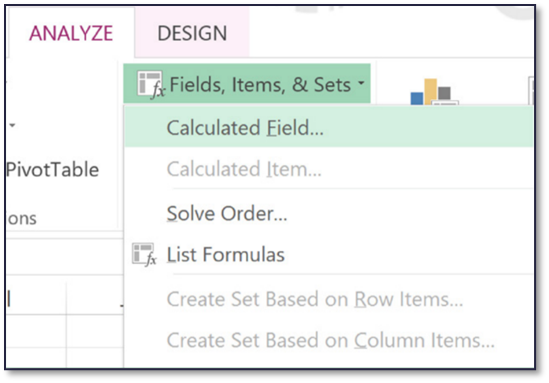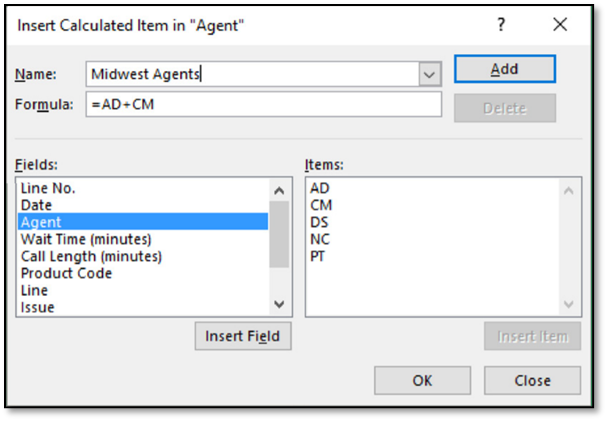# Pivot Tables: Working With Calculated Fields

Taylor Karl1166 0

A PivotTable is a data-processing tool used to query, organize, and summarize data or information between spreadsheets, tables, or databases. Drag and drop fields into a PivotTable toPart One: Working with Calculated Fields

What are Calculated Fields?

Calculated Fields are formulas that can refer to other fields in the pivot table. Before you begin, decide whether you want a calculated field or a calculated item within a field. Use a calculated item when you want your formula to use data from one or more specific items within a field. Use a calculated field when you want to use the data from another field in your formula. You could potentially use a calculated field to:

• calculate a bonus for sales reps based on their sales
• calculate average sales for the quarter based on revenue

1. Click in a cell in the PivotTable.

2. Click PivotTable Tools → Analyze → Calculations → Fields, Items, & Sets → Calculated Field.

3. In the Name box, type a name for the calculated field.

4. In the Formula box, type the desired formula. To use data from one of the PivotTable fields, click the desired field in the Fields box, and then click Insert Field. For example, to calculate a 15% commission on each value in the Sales field, enter:

=Sales*15%

5. Click Add to save the calculated field and click OK.

6. The new field appears in the Values area of the PivotTable as well as in the field list in the PivotTable Field List.Deleting a Calculated Field

1. Click a cell in the PivotTable.

2. Click PivotTable Tools → Analyze → Calculations → Fields, Items,

& Sets → Calculated Field.

3. In the Name box, click the drop-down arrow.

4. Select the field to delete.

5. Click Delete.

6. Click Close.

1. Click on one of the existing items in the field of the PivotTable.

Note: Users must have one of the existing items in the field selected. The

option for the calculated item will be grayed out if the field is not selected.

2. Click PivotTable Tools → Analyze → Calculations → Fields, Items, & Sets → Calculated Item.

3. In the Name box, type a name for the calculated item.

4. In the Formula box, type the desired formula. Click the desired item in the Items box and click Insert Item to include the item in the formula. For example:

=Jan+Feb+Mar

5. Click Add to save the calculated item and click OK.

6. The new calculated item appears in the PivotTable. It is also available in the filter pull-down menu for that field.

Note: Be careful when using calculated items when grand totals are

present. The calculated item may be double-counting other field items,

which can create inaccurate grand totals.Deleting a Calculated Item

1. Click on one of the cells in the field that contains the Calculated Item.

2. Click PivotTable Tools → Analyze → Calculations → Fields, Items,

& Sets → Calculated Item.

3. In the Name box, click the drop-down arrow.

4. Select the item to delete.

5. Click Delete.

6. Click Close.

Print